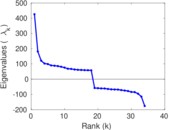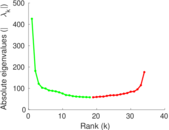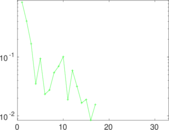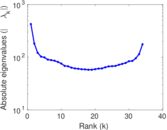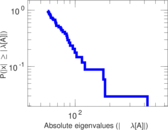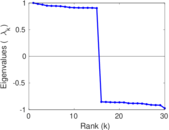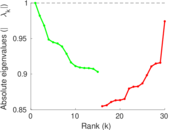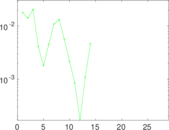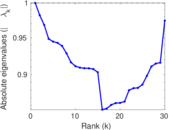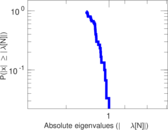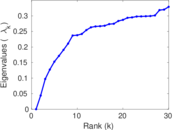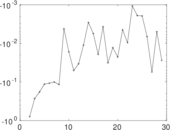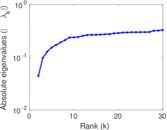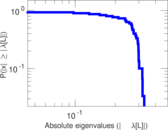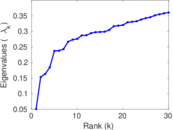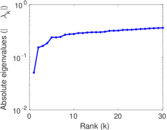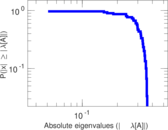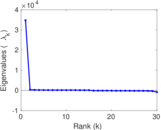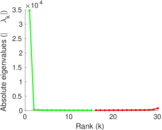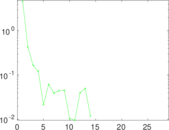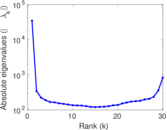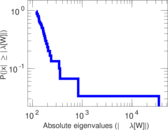# Internet topology

This is the network of connections between autonomous systems of the Internet. The nodes are autonomous systems (AS), i.e. collections of connected IP routing prefixes controlled by independent network operators. Edges are connections between autonomous systems. Multiple edges may connect two nodes, each representing an individual connection in time. Edges are annotated with the timepoint of the connection.

 Code `TO` Internal name `topology` Name Internet topology Data source http://irl.cs.ucla.edu/topology/ AvailabilityDataset is available for download Consistency checkDataset passed all tests Category Computer network Node meaning Autonomous system Edge meaning Connection Network format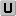Unipartite, undirected Edge typeUnweighted, multiple edges Temporal dataEdges are annotated with timestamps LoopsDoes not contain loops Orientation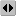Is not directed, but the underlying data is

## Statistics

 Size n = 34,761 Volume m = 171,403 Unique edge count m̿ = 114,496 Loop count l = 0 Wedge count s = 34,304,301 Claw count z = 20,603,857,222 Cross count x = 10,997,020,148,941 Triangle count t = 554,749 Square count q = 79,912,964 4-Tour count T4 = 776,736,356 Maximum degree dmax = 5,305 Average degree d = 9.861 80 Fill p = 0.000 189 517 Average edge multiplicity m̃ = 1.497 02 Size of LCC N = 34,761 Diameter δ = 10 50-Percentile effective diameter δ0.5 = 3.304 83 90-Percentile effective diameter δ0.9 = 4.372 41 Median distance δM = 4 Mean distance δm = 3.783 62 Gini coefficient G = 0.808 028 Balanced inequality ratio P = 0.164 131 Relative edge distribution entropy Her = 0.807 662 Power law exponent γ = 2.233 38 Tail power law exponent γt = 1.921 00 Tail power law exponent with p γ3 = 1.921 00 p-value p = 0.000 00 Degree assortativity ρ = −0.214 867 Degree assortativity p-value pρ = 0.000 00 Clustering coefficient c = 0.048 514 2 Spectral norm α = 425.817 Algebraic connectivity a = 0.044 087 6 Spectral separation |λ1[A] / λ2[A]| = 2.341 89 Non-bipartivity bA = 0.586 838 Normalized non-bipartivity bN = 0.016 724 9 Algebraic non-bipartivity χ = 0.032 772 5 Spectral bipartite frustration bK = 0.001 321 95 Controllability C = 24,439 Relative controllability Cr = 0.703 058

## Plots

### Fruchterman–Reingold graph drawing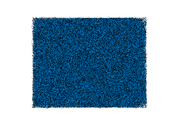### Degree distribution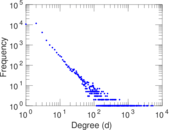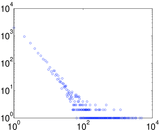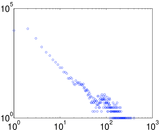### Cumulative degree distribution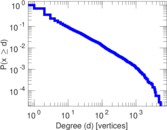### Lorenz curve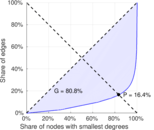### Spectral distribution of the adjacency matrix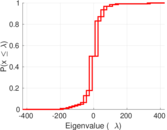### Spectral distribution of the normalized adjacency matrix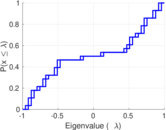### Spectral distribution of the Laplacian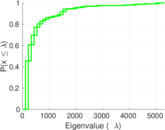### Spectral graph drawing based on the adjacency matrix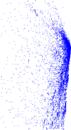### Spectral graph drawing based on the Laplacian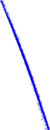### Spectral graph drawing based on the normalized adjacency matrix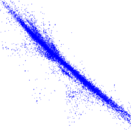### Degree assortativity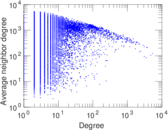### Zipf plot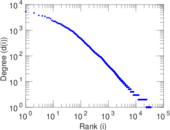### Hop distribution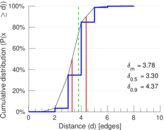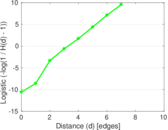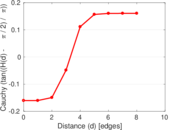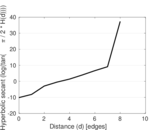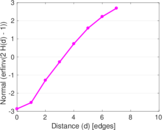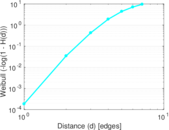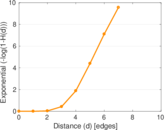### Double Laplacian graph drawing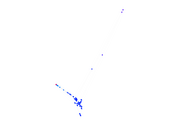### Delaunay graph drawing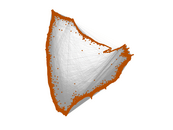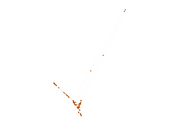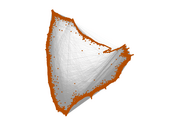### Edge weight/multiplicity distribution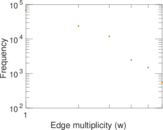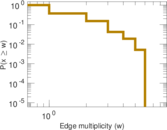### Clustering coefficient distribution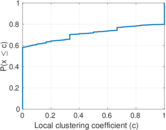### Average neighbor degree distribution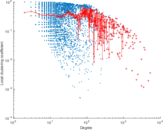### Temporal distribution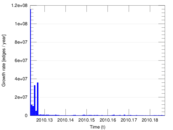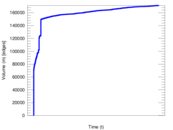### Temporal hop distribution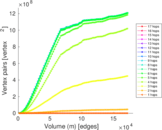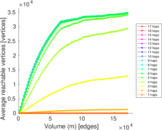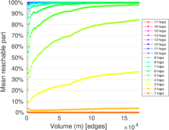### Diameter/density evolution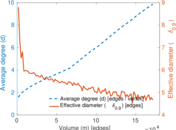### SynGraphy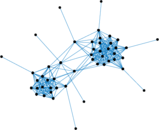### Inter-event distribution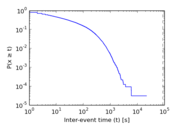### Node-level inter-event distribution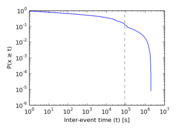### Matrix decompositions plots